Courses

# Test: Mental Ability, Analytical & Logical Reasoning - 12 (2011-2010)

## 15 Questions MCQ Test UPSC Topic Wise Previous Year Questions | Test: Mental Ability, Analytical & Logical Reasoning - 12 (2011-2010)

Description
This mock test of Test: Mental Ability, Analytical & Logical Reasoning - 12 (2011-2010) for UPSC helps you for every UPSC entrance exam. This contains 15 Multiple Choice Questions for UPSC Test: Mental Ability, Analytical & Logical Reasoning - 12 (2011-2010) (mcq) to study with solutions a complete question bank. The solved questions answers in this Test: Mental Ability, Analytical & Logical Reasoning - 12 (2011-2010) quiz give you a good mix of easy questions and tough questions. UPSC students definitely take this Test: Mental Ability, Analytical & Logical Reasoning - 12 (2011-2010) exercise for a better result in the exam. You can find other Test: Mental Ability, Analytical & Logical Reasoning - 12 (2011-2010) extra questions, long questions & short questions for UPSC on EduRev as well by searching above.
QUESTION: 1

### Consider the following argument : "In order to be a teacher one must graduate from college. All poets are poor. Some Mathematicians are poets. No college graduate is poor." Which one of the following is not a valid conclusion regarding the above argument? 

Solution:

We can draw the following two diagrams from given arguments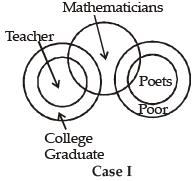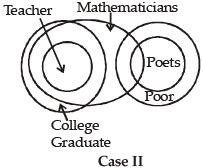From case II, the option (b) is not a valid conclusion.

QUESTION: 2

### Consider the following figure and answer the item that follows: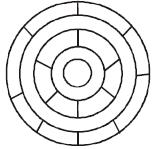What is the minimum number of different colours required to paint the figure given above such that no two adjacent regions have the same colour? 

Solution:

Suppose R, B and G are three different colours to paint the following figure.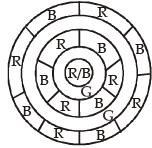Thus, there will be three different colours required to paint the figure at the given conditions.

QUESTION: 3

### There are four routes to travel from city A to city B and six routes from city B to city C. How many routes are possible to travel from the city A to city C? 

Solution:

Total number of possible routes from the city A to city C = 4 x 6 = 24

QUESTION: 4

Each of the following two items consists of four statements. Of these four statements, two cannot both be true, but both can be false. Study the statements carefully and identify the two that satisfy the above condition. Select the correct answer using the codes given below each set of statements:

Q. Examine the following statements :
1. All animals are carnivorous.
2. Some animals are not carnivorous.
3. Animals are not carnivorous.
4. Some animals are carnivorous.



Solution:

Statements 1 and 3 contradict each-other, hence both statements cannot be true together. But there is a situation where in both statements are false that is if some of the animals are carnivorous and some are not.

QUESTION: 5

Each of the following two items consists of four statements. Of these four statements, two cannot both be true, but both can be false. Study the statements carefully and identify the two that satisfy the above condition. Select the correct answer using the codes given below each set of statements:

Q. Examine the following statements :
1. All trains are run by diesel engine.
2. Some trains are run by diesel engine.
3. No train is run by diesel engine.
4. Some trains are not run by diesel engine.



Solution:

Statement 1 and 3 contradict each-other, hence both statements cannot be true together. But there are a situation where in both statements are false that is if some of the trains are run on diesel engine and some are not.

QUESTION: 6

In a queue, Mr. X is fourteenth from the front and Mr. Y is seventeenth from the end, while Mr. Z is exactly in between Mr. X and Mr. Y. If Mr. X is ahead of Mr. Y and there are 48 persons in the queue, how many persons are there between Mr. X and Mr. Z?



Solution:

Total number of persons in the queue = 48
Position of Mr. X from the front = 14
Position of Mr. Y from the end = 17
∴  Position of Mr. Y from the front = 48 – 17 + 1= 32
Rank of  Mr. Z from the front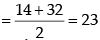Thus total number of persons between Mr. X and Mr. Z = 23 – 14 – 1 = 8

QUESTION: 7

In a survey regarding a proposed measure to be introduced, 2878 persons took part of which 1652 were males. 1226 persons voted against the proposal of which 796 were males. 1425 persons voted for the proposal. 196 females were undecided.

Q. How many females voted for the proposal?



Solution: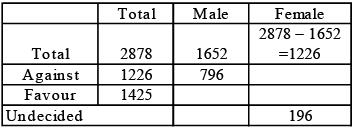Now, number of female voted against = 1226 –796 = 430
Now, number of female voted for the proposal = 1226 – (430 + 196) = 1226 – 626 = 600
No. of undecided = 2878 – (1226 + 1425) = 227
Now, No. of undecided male = 227 – 196 = 31

QUESTION: 8

In a survey regarding a proposed measure to be introduced, 2878 persons took part of which 1652 were males. 1226 persons voted against the proposal of which 796 were males. 1425 persons voted for the proposal. 196 females were undecided.

Q. How many males were undecided?



Solution:Now, number of female voted against = 1226 –796 = 430
Now, number of female voted for the proposal = 1226 – (430 + 196) = 1226 – 626 = 600
No. of undecided = 2878 – (1226 + 1425) = 227
Now, No. of undecided male = 227 – 196 = 31

QUESTION: 9

In a survey regarding a proposed measure to be introduced, 2878 persons took part of which 1652 were males. 1226 persons voted against the proposal of which 796 were males. 1425 persons voted for the proposal. 196 females were undecided.

Q. How many females were not in favour of the proposal?



Solution:Now, number of female voted against = 1226 –796 = 430
Now, number of female voted for the proposal = 1226 – (430 + 196) = 1226 – 626 = 600
No. of undecided = 2878 – (1226 + 1425) = 227
Now, No. of undecided male = 227 – 196 = 31

QUESTION: 10

A, B, C, D and E are members of the same family. There are two fathers, two sons, two wives, three males and two females. The teacher was the wife of a lawyer who was the son of a doctor. E is not a male, neither also a wife of a professional. C is the youngest person in the family and D is the eldest. B is a male.

Q. How is D related to E?



Solution:

The given information can be tabulated as follows: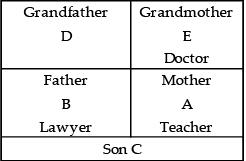QUESTION: 11

A, B, C, D and E are members of the same family. There are two fathers, two sons, two wives, three males and two females. The teacher was the wife of a lawyer who was the son of a doctor. E is not a male, neither also a wife of a professional. C is the youngest person in the family and D is the eldest. B is a male.

Q. Who are the females in the group?



Solution:

The given information can be tabulated as follows:QUESTION: 12

A, B, C, D and E are members of the same family. There are two fathers, two sons, two wives, three males and two females. The teacher was the wife of a lawyer who was the son of a doctor. E is not a male, neither also a wife of a professional. C is the youngest person in the family and D is the eldest. B is a male.

Q. Whose wife is the teacher?



Solution:

The given information can be tabulated as follows:QUESTION: 13

A cuboid has six sides of different colours. The red side is opposite to black. The blue side is adjacent to white. The brown side is adjacent to blue. The red side is face down. Which one of the following would be the opposite to brown?



Solution:

Here, figure is drawn on the basis of the given conditions. White and brown are on the opposite faces.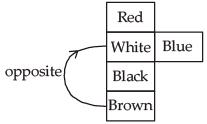QUESTION: 14

In a meeting, the map of a village was placed in such a manner that south-east becomes north, north-east becomes west and so on. What will south become?



Solution: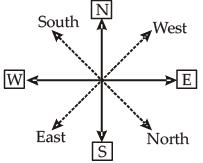From the figure, it is clear that ‘S’ becomes ‘North-east’ in the new figure (dotted line)

QUESTION: 15

P, Q, R and S are four men. P is the oldest but not the poorest. R is the richest but not the oldest. Q is older than S but not than P or R. P is richer than Q but not than S. The four men can be ordered (descending) in respect of age and richness, respectively, as



Solution:

Q > S, P > Q, R > Q
Age: As, Q > S, P > Q, R > Q
Also, P is the oldest.
∴ P > R > Q > S
Richness : As, P > Q, S > P
Also R is he richest.
∴ R > S > P > Q